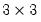cov
Compute covariances, correlations and other measures of association for the series in a group.
You may compute measures related to Pearson product-moment (ordinary) covariances and correlations, Spearman rank covariances, or Kendall’s tau along with test statistics for evaluating whether the correlations are equal to zero.
Syntax
group_name.cov(options) [keywords [@partial z1 z2 z3...]]
You should specify keywords indicating the statistics you wish to display from the list below, optionally followed by the keyword @partial and a list of conditioning series or groups (for the group view), or the name of a conditioning matrix (for the matrix view).
You may specify keywords from one of the four sets (Pearson correlation, Spearman correlation, Kendall’s tau, Uncentered Pearson) corresponding the computational method you wish to employ. (You may not select keywords from more than one set.)
If you do not specify keywords, EViews will assume “cov” and compute the Pearson covariance matrix. Note that Group::cov is equivalent to the Group::cor command with a different default setting.
Pearson Correlation

 cov Product moment covariance. corr Product moment correlation. sscp Sums-of-squared cross-products. stat Test statistic (t-statistic) for evaluating whether the correlation is zero. prob Probability under the null for the test statistic. cases Number of cases. obs Number of observations. wgts Sum of the weights.
Spearman Rank Correlation

 rcov Spearman’s rank covariance. rcorr Spearman’s rank correlation. rsscp Sums-of-squared cross-products. rstat Test statistic (t-statistic) for evaluating whether the correlation is zero. rprob Probability under the null for the test statistic. cases Number of cases. obs Number of observations. wgts Sum of the weights.
Kendall’s tau

 taub Kendall’s tau-b. taua Kendall’s tau-a. taucd Kendall’s concordances and discordances. taustat Kendall’s score statistic for evaluating whether the Kendall’s tau-b measure is zero. tauprob Probability under the null for the score statistic. cases Number of cases. obs Number of observations. wgts Sum of the weights.
Uncentered Pearson

 ucov Product moment covariance. ucorr Product moment correlation. usscp Sums-of-squared cross-products. ustat Test statistic (t-statistic) for evaluating whether the correlation is zero. uprob Probability under the null for the test statistic. cases Number of cases. obs Number of observations. wgts Sum of the weights.
Note that cases, obs, and wgts are available for each of the methods.

Options

 wgt=name (optional) Name of series containing weights. wgtmethod=arg (default = “sstdev” Weighting method (when weights are specified using “weight=”): frequency (“freq”), inverse of variances (“var”), inverse of standard deviation (“stdev”), scaled inverse of variances (“svar”), scaled inverse of standard deviations (“sstdev”).Only applicable for ordinary (Pearson) calculations. Weights specified by “wgt=” are frequency weights for rank correlation and Kendall’s tau calculations. pairwise Compute using pairwise deletion of observations with missing cases (pairwise samples). df Compute covariances with a degree-of-freedom correction to account for estimated means (for centered specifications), and any partial conditioning variables. multi=arg (default=“none”) Adjustment to p-values for multiple comparisons: none (“none”), Bonferroni (“bonferroni”), Dunn-Sidak (“dunn”). outfmt=arg (default= “single”) Output format: single table (“single”), multiple table (“mult”), list (“list”), spreadsheet (“sheet”). Note that “outfmt=sheet” is only applicable if you specify a single statistic keyword. out=name Basename for saving output. All results will be saved in Sym matrices named using keys (“COV”, “CORR”, “SSCP”, “TAUA”, “TAUB”, “CONC” (Kendall’s concurrences), “DISC” (Kendall’s discordances), “CASES”, “OBS”, “WGTS”) appended to the basename (e.g., the covariance specified by “out=my” is saved in the Sym matrix “MYCOV”). prompt Force the dialog to appear from within a program. p Print the result.
Examples
group grp1 height weight age
grp1.cov
displays aPearson covariance matrix for the three series in GRP1.
grp1.cov corr stat prob
displays a table containing the Pearson correlation, t-statistic for testing for zero correlation, and associated p-value, for the series in GRP1.
grp1.cov(pairwise) taub taustat tauprob
computes the Kendall’s tau-b, score statistic, and p-value for the score statistic, using samples with pairwise missing value exclusion.
grp1.cov(out=aa) cor @partial gender
computes the Pearson correlation for the series in GRP1 conditional on GENDER and saves the results in the symmetric matrix object AACORR.
Cross-references
See also Group::cor. For simple forms of the calculation, see @cor, and @cov.